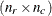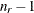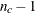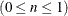### PROC CORRESP Statement

PROC CORRESP <options> ;

The PROC CORRESP statement invokes the CORRESP procedure. Table 32.1 summarizes the options available in the PROC CORRESP statement. These options are described following the table.

Table 32.1: Summary of PROC CORRESP Statement Options

Option

Description

Data Set Options

Specifies input SAS data set

Specifies output coordinate SAS data set

Specifies output frequency SAS data set

Row and Column Coordinates

Specifies the number of dimensions or axes

Performs multiple correspondence analysis

Standardizes the row and column coordinates

Table Construction

Specifies binary table

Specifies cross levels of TABLES variables

Specifies input data in PROC FREQ output

Includes observations with missing values

Control Displayed Output

Displays all output

Displays inertias adjusted by Benzécri’s method

Displays cell contributions to chi-square

Displays column profile matrix

Displays observed minus expected values

Displays chi-square expected values

Displays inertias adjusted by Greenacre’s method

Suppresses the display of column coordinates

Suppresses the display of all output

Suppresses the display of row coordinates

Displays contingency table of observed frequencies

Specifies ODS Graphics details

Displays percentages or frequencies

Displays row profile matrix

Suppresses all point and coordinate statistics

Other Options

Specifies esoteric column coordinate standardizations

Specifies minimum inertia

Specifies number of classification variables

Specifies esoteric row coordinate standardizations

Specifies effective zero

Includes level source in the OUTC= data set

The display options control the amount of displayed output. The CELLCHI2, EXPECTED, and DEVIATION options display additional chi-square information. See the Details: CORRESP Procedure section for more information. The unit of the matrices displayed by the CELLCHI2, CP, DEVIATION, EXPECTED, OBSERVED, and RP options depends on the value of the PRINT= option. The table construction options control the construction of the contingency table; these options are valid only when you also specify a TABLES statement.

You can specify the following options in the PROC CORRESP statement. They are given in alphabetical order.

ALL

is equivalent to specifying the OBSERVED, RP, CP, CELLCHI2, EXPECTED, and DEVIATION options. Specifying the ALL option does not affect the PRINT= option. Therefore, only frequencies (not percentages) for these options are displayed unless you specify otherwise with the PRINT= option.

BENZECRI
BEN

displays adjusted inertias when performing multiple correspondence analysis. By default, unadjusted inertias, the usual inertias from multiple correspondence analysis, are displayed. However, adjusted inertias that use a method proposed by Benzécri (1979) and described by Greenacre (1984, p. 145) can be displayed by specifying the BENZECRI option. Specify the UNADJUSTED option to output the usual table of unadjusted inertias as well. See the section MCA Adjusted Inertias for more information.

BINARY

enables you to create binary tables easily. When you specify the BINARY option, specify only column variables in the TABLES statement. Each input data set observation forms a single row in the constructed table.

CELLCHI2
CEL

displays the contribution to the total chi-square test statistic for each cell. See also the descriptions of the DEVIATION, EXPECTED, and OBSERVED options.

COLUMN=B | BD | DB | DBD | DBD1/2 | DBID1/2
COL=B | BD | DB | DBD | DBD1/2 | DBID1/2

provides other standardizations of the column coordinates. The COLUMN= option is rarely needed. Typically, you should use the PROFILE= option instead (see the section The PROFILE=, ROW=, and COLUMN= Options). By default, COLUMN=DBD.

CP

displays the column profile matrix. Column profiles contain the observed conditional probabilities of row membership given column membership. See also the RP option.

CROSS=BOTH | COLUMN | NONE | ROW
CRO=BOT | COL | NON | ROW

specifies the method of crossing (factorially combining) the levels of the TABLES variables. The default is CROSS=NONE.

NONE

causes each level of every row variable to become a row label and each level of every column variable to become a column label.

ROW

causes each combination of levels for all row variables to become a row label, whereas each level of every column variable becomes a column label.

COLUMN

causes each combination of levels for all column variables to become a column label, whereas each level of every row variable becomes a row label.

BOTH

causes each combination of levels for all row variables to become a row label and each combination of levels for all column variables to become a column label.

The section TABLES Statement provides a more detailed description of this option.

DATA=SAS-data-set

specifies the SAS data set to be used by PROC CORRESP. If you do not specify the DATA= option, PROC CORRESP uses the most recently created SAS data set.

DEVIATION
DEV

displays the matrix of deviations between the observed frequency matrix and the product of its row marginals and column marginals divided by its grand frequency. For ordinary two-way contingency tables, these are the observed minus expected frequencies under the hypothesis of row and column independence and are components of the chi-square test statistic. See also the CELLCHI2, EXPECTED, and OBSERVED options.

DIMENS=n
DIM=n

specifies the number of dimensions or axes to use. The default is DIMENS=2. The maximum value of the DIMENS= option in antable isor, whichever is smaller. For example, in a table with 4 rows and 5 columns, the maximum specification is DIMENS=3. If your table has 2 rows or 2 columns, specify DIMENS=1.

EXPECTED
EXP

displays the product of the row marginals and the column marginals divided by the grand frequency of the observed frequency table. For ordinary two-way contingency tables, these are the expected frequencies under the hypothesis of row and column independence and are components of the chi-square test statistic. In other situations, this interpretation is not strictly valid. See also the CELLCHI2, DEVIATION, and OBSERVED options.

FREQOUT
FRE

indicates that the PROC CORRESP input data set has the same form as an output data set from the FREQ procedure, even if it was not directly produced by PROC FREQ. The FREQOUT option enables PROC CORRESP to take shortcuts in constructing the contingency table.

When you specify the FREQOUT option, you must also specify a WEIGHT statement. The cell frequencies in a PROC FREQ output data set are contained in a variable called COUNT, so specify COUNT in a WEIGHT statement with PROC CORRESP. The FREQOUT option might produce unexpected results if the DATA= data set is structured incorrectly. Each of the two variable lists specified in the TABLES statement must consist of a single variable, and observations must be grouped by the levels of the row variable and then by the levels of the column variable. It is not required that the observations be sorted by the row variable and column variable, but they must be grouped consistently. There must be as many observations in the input data set (or BY group) as there are cells in the completed contingency table. Zero cells must be specified with zero weights. When you use PROC FREQ to create the PROC CORRESP input data set, you must specify the SPARSE option in the FREQ procedure’s TABLES statement so that the zero cells are written to the output data set.

GREENACRE
GRE

displays adjusted inertias when you are performing multiple correspondence analysis. By default, unadjusted inertias, the usual inertias from multiple correspondence analysis, are displayed. However, adjusted inertias that use a method proposed by Greenacre (1984, p. 156) can be displayed by specifying the GREENACRE option. Specify the UNADJUSTED option to output the usual table of unadjusted inertias as well. See the section MCA Adjusted Inertias for more information.

MCA

requests a multiple correspondence analysis. This option requires that the input table be a Burt table, which is a symmetric matrix of crosstabulations among several categorical variables. If you specify the MCA option and a VAR statement, you must also specify the NVARS= option, which gives the number of categorical variables that were used to create the table. With raw categorical data, if you want results for the individuals as well as the categories, use the BINARY option instead.

MININERTIA=n
MIN=n

specifies the minimum inertiaused to create the best tables—the indicator of which points best explain the inertia of each dimension. By default, MININERTIA=0.8. See the section Algorithm and Notation for more information.

MISSING
MIS

specifies that observations with missing values for the TABLES statement variables are included in the analysis. Missing values are treated as a distinct level of each categorical variable. By default, observations with missing values are excluded from the analysis.

NOCOLUMN <= BOTH | DATA | PRINT>
NOC <= BOT | DAT | PRI>

suppresses the display of the column coordinates and statistics and omits them from the output coordinate data set.

BOTH

suppresses all column information from both the SAS listing and the output data set. The NOCOLUMN option is equivalent to the option NOCOLUMN=BOTH.

DATA

suppresses all column information from the output data set.

PRINT

suppresses all column information from the SAS listing.

NOPRINT
NOP

suppresses the display of all output. This option is useful when you need only an output data set. This option disables the Output Delivery System (ODS), including ODS Graphics, for the duration of the PROC. For more information, see Chapter 20: Using the Output Delivery System.

NOROW <= BOTH | DATA | PRINT>
NOR <= BOT | DAT | PRI>

suppresses the display of the row coordinates and statistics and omits them from the output coordinate data set.

BOTH

suppresses all row information from both the SAS listing and the output data set. The NOROW option is equivalent to the option NOROW=BOTH.

DATA

suppresses all row information from the output data set.

PRINT

suppresses all row information from the SAS listing.

The NOROW option can be useful when the rows of the contingency table are replications.

NVARS=n
NVA=n

specifies the number of classification variables that were used to create the Burt table. For example, suppose the Burt table was originally created with the following statement:

tables a b c;


You must specify NVARS=3 to read the table with a VAR statement.

The NVARS= option is required when you specify both the MCA option and a VAR statement. (See the section VAR Statement for an example.)

OBSERVED
OBS

displays the contingency table of observed frequencies and its row, column, and grand totals. If you do not specify the OBSERVED or ALL option, the contingency table is not displayed.

OUTC=SAS-data-set
OUT=SAS-data-set

creates an output coordinate SAS data set to contain the row, column, supplementary observation, and supplementary variable coordinates. This data set also contains the masses, squared cosines, quality of each point’s representation in the DIMENS=n dimensional display, relative inertias, partial contributions to inertia, and best indicators.

OUTF=SAS-data-set

creates an output frequency SAS data set to contain the contingency table, row, and column profiles, the expected values, and the observed minus expected values and contributions to the chi-square statistic.

PLOTS<(global-plot-options)> <= plot-request <(options)>>
PLOTS<(global-plot-options)> <= (plot-request <(options)> <... plot-request <(options)>>)>

specifies options that control the details of the plots. When you specify only one plot request, you can omit the parentheses around the plot request.

ODS Graphics must be enabled before plots can be requested. For example:

ods graphics on;

proc corresp;
tables Marital, Origin;
run;

ods graphics off;


For more information about enabling and disabling ODS Graphics, see the section Enabling and Disabling ODS Graphics in Chapter 21: Statistical Graphics Using ODS.

By default, for simple correspondence analysis, PROC CORRESP prints the configuration of points consisting of the row coordinates and column coordinates. With MCA, only column coordinates are printed. The default plots (y * x) are Dim2 * Dim1, Dim3 * Dim1, Dim3 * Dim2, and so on. When you specify PLOTS(FLIP), the plots are Dim1 * Dim2, Dim1 * Dim3, Dim2 * Dim3, and so on.

The global plot option is as follows:

FLIP
FLI

flips or interchanges the X-axis and Y-axis dimensions.

The plot requests include the following:

ALL

produces all appropriate plots.

NONE
NON

suppresses all plots.

PRINT=BOTH | FREQ | PERCENT
PRI=BOT | FRE | PER

affects the OBSERVED, RP, CP, CELLCHI2, EXPECTED, and DEVIATION options. The default is PRINT=FREQ.

• The PRINT=FREQ option displays output in the appropriate raw or natural units. (That is, PROC CORRESP displays raw frequencies for the OBSERVED option, relative frequencies with row marginals of 1.0 for the RP option, and so on.)

• The PRINT=PERCENT option scales results to percentages for the display of the output. (All elements in the OBSERVED matrix sum to 100.0, the row marginals are 100.0 for the RP option, and so on.)

• The PRINT=BOTH option displays both percentages and frequencies.

PROFILE=BOTH | COLUMN | NONE | ROW
PRO=BOT | COL | NON | ROW

specifies the standardization for the row and column coordinates. The default is PROFILE=BOTH.

BOTH

specifies a standard correspondence analysis, which jointly displays the principal row and column coordinates. Row coordinates are computed from the row profile matrix, and column coordinates are computed from the column profile matrix.

ROW

specifies a correspondence analysis of the row profile matrix. The row coordinates are weighted centroids of the column coordinates.

COLUMN

specifies a correspondence analysis of the column profile matrix. The column coordinates are weighted centroids of the row coordinates.

NONE

is rarely needed. Row and column coordinates are the generalized singular vectors, without the customary standardizations.

provides other standardizations of the row coordinates. The ROW= option is rarely needed. Typically, you should use the PROFILE= option instead (see the section The PROFILE=, ROW=, and COLUMN= Options). By default, ROW=DAD.

RP

displays the row profile matrix. Row profiles contain the observed conditional probabilities of column membership given row membership. See also the CP option.

SHORT
SHO

suppresses the display of all point and coordinate statistics except the coordinates. The following information is suppressed: each point’s mass, relative contribution to the total inertia, and quality of representation in the DIMENS=n dimensional display; the squared cosines of the angles between each axis and a vector from the origin to the point; the partial contributions of each point to the inertia of each dimension; and the best indicators.

SINGULAR=n
SIN=n

specifies the largest value that is considered to be within rounding error of zero. The default value is 1E–8. This parameter is used in checking for zero rows and columns, in checking Burt table diagonal sums for equality, in checking denominators before dividing, and so on. Typically, you should not assign a value outside the range 1E–6 to 1E–12.

SOURCE
SOU

adds the variable _VAR_, which contains the name or label of the variable corresponding to the current level, to the OUTC= and OUTF= data sets.